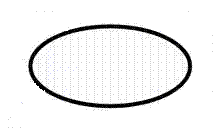### Ellipse Calculator

An ellipse is the set of all points in a plane such that the sum of the distances from two fixed points to every point in line is constant. The formula generally associated with the focus of an ellipse is c2= a2 − b2 where c is the distance from the focus to vertex and b is the distance from the vertex a co-vetex on the minor axisArea of Ellipse : [ π×r1×r2 ] Please enter the equatorial radii = The Area of Ellipse =The Volume of Ellipse : [ (4/3)×π×r1×r2×r3 ] Please enter the equatorial radii = The Volume of Ellipse =Perimeter of Ellipse : [ 2×π×Sqrt((r1² + r2²)/2) ] Pease enter the equatorial radii = Perimeter of Ellipse =